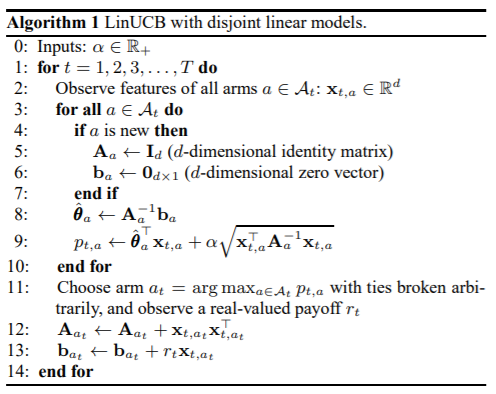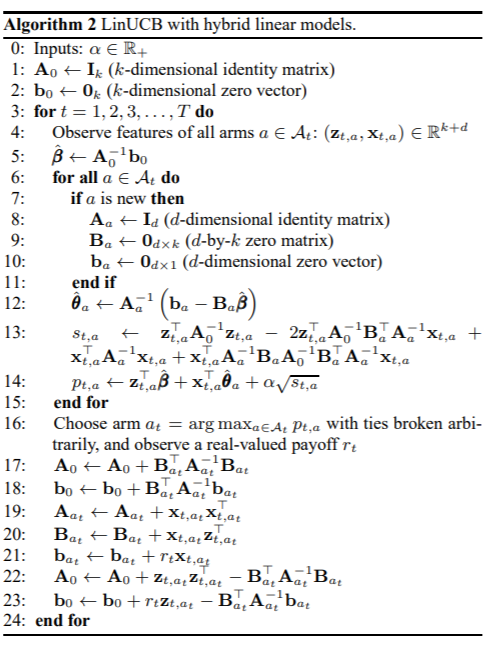# 强化学习基础——bandit

ε-Greedy算法

Thompson sampling算法

Thompson sampling算法流程

UCB

UCB算法流程

LinUCB

Context Bandit-附加信息刻画决策过程

Context Bandit如何学习

LinUCB算法流程

LinUCB代码

LinUCB with Hybrid Linear Models

Hybrid LinUCB算法流程

Hybrid LinUCB代码

# 为选择而生

Exploration的代价是要不停的试错，试错成本高，但有助于更加准确的估计选择的概率；
Exploitation会基于目前的估计拿出“最好的”选择，但目前的估计可能是不准的（因为试错依然不够多）。

# 为错误买单代价

Bandit算法需要量化一个核心问题：探索成本有多大？能不能少为错误买单？

# 经典方法

## ε-Greedy算法

• 概率选择探索，并根据反馈更新概率
• 概率选择利用，从中选择概率最高的做决策；

## Thompson sampling算法

good choice概率是一个客观存在的、固定的值，可以用一个概率分布来描述的不确定性。随着样本的增加，这个概率分布在真实附近的概率密度会越来越大。

Bayesian学派会用概率分布来描述不确定性：

• 如果的结果为1，则会得到
• 如果的结果为0，则会得到

beta分布可以看作一个概率的概率分布，对二项分布中成功概率p的概率分布的描述。

### Thompson sampling算法流程

• 刻画good choice的概率，得到
• 对每个choice 随机抽取一个样本，得到
• 推荐最大的choice，得到
• 更新的分布：

## UCB

item被试错k次，计算item选择概率：

### UCB算法流程

• 考虑了回报均值的不确定性，让新的item更快得到尝试机会，将探索+开发融为一体；
• 基础的UCB算法不需要任何参数，因此不需要考虑如何验证参数（ε如何确定）的问题；

UCB算法的缺点：

• UCB算法需要首先尝试一遍所有item，因此当item数量很多时是一个问题；
• 一开始各item选择次数都比较少，导致得到的回报波动较大（经常选中实际比较差的item）；

## LinUCB

Yahoo!的科学家们在2010年发表了A Contextual-Bandit Approach to Personalized News Article Recommendation，利用context信息刻画决策过程，称之为LinUCB。

### Context Bandit-附加信息刻画决策过程

• 观测到特征向量x=(item类型，item标签，男，女，年龄，时间，地域)；
• 预测用户是否选择：，其中是要学习系数；
• 对所有item进行预测得到选择；

### LinUCB算法流程### LinUCB代码

def update(reward):
if reward == -1:
return
elif reward == 1:
r = r1
elif reward == 0:
r = r2

Aa[max_a] += np.outer(x, x)
Aa_inv[max_a] = np.linalg.inv(Aa[max_a])
ba[max_a] += r * x
theta[max_a] = Aa_inv[max_a].dot(ba[max_a])

def recommend(time, user_features, choices):
global max_a
global x

article_len = len(choices)

x = np.array(user_features).reshape((d, 1))
x_t = np.transpose(x)
index = [index_all[article] for article in choices]
UCB = np.matmul(np.transpose(theta[index], (0, 2, 1)), x) + alpha * np.sqrt(np.matmul(x_t, Aa_inv[index].dot(x)))

max_index = np.argmax(UCB)
max_a = index[max_index]
return choices[max_index]


### Hybrid LinUCB算法流程### Hybrid LinUCB代码

    def update(self, reward):
if reward == -1:
pass
elif reward == 1 or reward == 0:
if reward == 1:
r = self.r1
else:
r = self.r0

self.A0 += self.BaT[self.a_max].dot(self.AaIBa[self.a_max])
self.b0 += self.BaT[self.a_max].dot(self.AaIba[self.a_max])
self.Aa[self.a_max] += np.dot(self.xa, self.xaT)
self.AaI[self.a_max] = np.linalg.inv(self.Aa[self.a_max])
self.Ba[self.a_max] += np.dot(self.xa, self.zT)
self.BaT[self.a_max] = np.transpose(self.Ba[self.a_max])
self.ba[self.a_max] += r * self.xa
self.AaIba[self.a_max] = np.dot(self.AaI[self.a_max], self.ba[self.a_max])
self.AaIBa[self.a_max] = np.dot(self.AaI[self.a_max], self.Ba[self.a_max])

self.A0 += np.dot(self.z, self.zT) - np.dot(self.BaT[self.a_max], self.AaIBa[self.a_max])
self.b0 += r * self.z - np.dot(self.BaT[self.a_max], self.AaIba[self.a_max])
self.A0I = np.linalg.inv(self.A0)
self.A0IBaTAaI[self.a_max] = self.A0I.dot(self.BaT[self.a_max]).dot(self.AaI[self.a_max])
# self.AaIBaA0IBaTAaI[self.a_max] = np.matmul(self.AaIBa[self.a_max], self.A0IBaTAaI[self.a_max])
self.beta = np.dot(self.A0I, self.b0)
self.theta = self.AaIba - np.dot(self.AaIBa, self.beta)

else:
pass

def recommend(self, timestamp, user_features, articles):
article_len = len(articles)  # 20

self.xa = np.array(user_features).reshape((self.d, 1))  # (6,1)
self.xaT = np.transpose(self.xa)  # (1,6)

index = [self.index_all[article] for article in articles]
article_features_tmp = self.article_features[index]

# za : feature of current user/article combination, k*1
za = np.outer(article_features_tmp.reshape(-1), self.xa).reshape((article_len, self.k, 1))  # (20,36,1)
zaT = np.transpose(za, (0, 2, 1))  # (20,1,36)

A0Iza = np.matmul(self.A0I, za)  # (20,36,1)
A0IBaTAaIxa = np.matmul(self.A0IBaTAaI[index], self.xa)  # (20,36,1)
AaIxa = self.AaI[index].dot(self.xa)  # (20,6,1)
AaIBaA0IBaTAaIxa = np.matmul(self.AaIBa[index], A0IBaTAaIxa)  # (20,6,1)
# AaIBaA0IBaTAaIxa = np.matmul(self.AaIBaA0IBaTAaI[index], self.xa) # (20,6,1)

s = np.matmul(zaT, A0Iza - 2 * A0IBaTAaIxa) + np.matmul(self.xaT, AaIxa + AaIBaA0IBaTAaIxa)  # (20,1,1)
p = zaT.dot(self.beta) + np.matmul(self.xaT, self.theta[index]) + self.alpha * np.sqrt(s)  # (20,1,1)
# assert (s < 0).any() == False
# assert np.isnan(np.sqrt(s)).any() == False

# print A0Iza.shape, A0IBaTAaIxa.shape, AaIxa.shape, AaIBaA0IBaTAaIxa.shape, s.shape, p.shape (for debugging)
max_index = np.argmax(p)
self.z = za[max_index]
self.zT = zaT[max_index]
art_max = index[max_index]
self.a_max = art_max  # article index with largest UCB

return articles[max_index]


©️2019 CSDN 皮肤主题: 黑客帝国 设计师: 上身试试